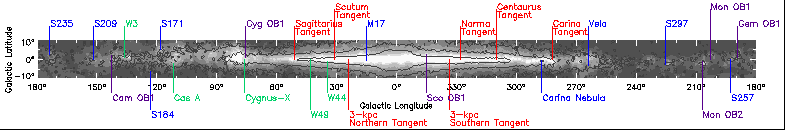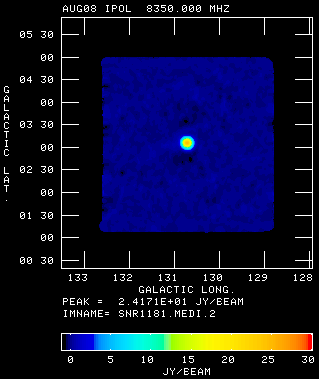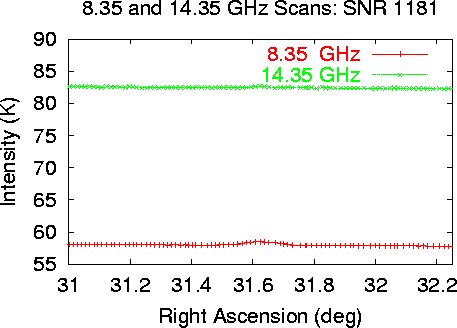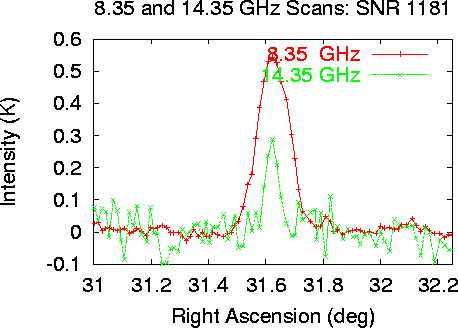# Introduction to Science in the Galactic Plane

## Lesson 9:The Calibrating Radio Data#QUESTION: How is Radio Telescope Data Calibrated?

After the radio measurements are made, they must be processed to distinguish true astronomical observations from effects due to the environment and equipment used in making the observations. The process of conversion and calibration is done in several steps. The goal is to convert to measurements to standard units so that observations made by different astronomers can be compared and scientific deductions made.

For the GPA survey, calibration is done in several steps. The first step is conversion is to convert from detector units of volts to measurements of the temperature. This is done by injecting a calibration signal of known temperature (measured in a laboratory) into the receiver. Then detector output is measured and the ratio of known temperature to detected output is used to convert Volts to Temperature.

After conversion to temperature calculations are made to fit a model of the sky and receiver contributions to the measurements.

The next calibration step is to determine the brightness temperature of a very compact radio source of known Flux Density (Janskys). Radio Astronomers use the unit Janskys to compare their results. One Jansky is 0.000000000000000000000000001 Watts/meter^2/Hz which is a very small value.

### Calibration Example for Supernova Remnant of year 1181 AD.This graph shows part of a single scan of the sky centered on SNR 1181. The X axis is the Right Ascension coordinate, which indicates where the telescope was pointing. The Y axis shows the total system temperature measured by each of two receivers, one with a center frequency of 8.35 GHz (3.6 centimeter wavelength, red) and 14.35 GHz (2.1 centimeter wavelength, green). Notice that there is only a very small variation in the system temperature measured along the scan. This is because astronomical object contribute a very small amount to the total detected power.
The major components of the measured brightness temperature are listed below:The sky brightness and receiver temperature components of the measurements are removed by fitting a curve to the ends of the measurements of brightness and subtracting this fit. The source SNR 1181 is clearly visible in these plots after removing the other effects. Notice that SNR 1181 is brighter at 8.35 GHz than 14.35 GHz. Also notice the noise in the scan is greater in the 14.35 GHz data. This is because the sky contribution to the 14.35 GHz data is much greater.After calibration, the measurements are averages and placed on a grid and a map of the region is produced, with units of Janskies. The final GPA maps are produced by averaging four independent images of the same regions of the sky. This process reduces the noise in the observations and provides a way of removing isolated errors in the images, such as bad weather.